Next: Orifice Up: Theory Previous: General   Contents

## Fluid Section Types: Gases

Before introducing the fluid section types for gases, a couple of fundamental aerodynamic equations are introduced. For details, the reader is referred to . The thermodynamic state of a gas is usually determined by the static pressure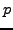, the static temperature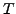and the density. For an ideal gas (the case considered here), they are related by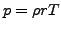(the ideal gas equation), where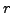is the specific gas constant.only depends on the material, it does not depend on the temperature.

The energy conservation law runs like :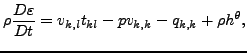(21)

where D denotes the total derivative. By use of the mass conservation: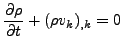(22)

and the conservation of momentum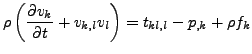(23)

this equation can also be written as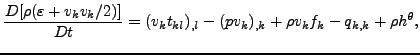(24)

or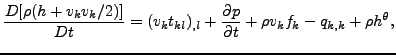(25)

where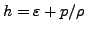is the entalpy. For an ideal gas one can write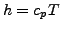,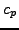is the heat capacity at constant pressure.

The total temperature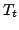is now defined as the temperature which is obtained by slowing down the fluid to zero velocity in an adiabatic way. Using the energy equation (25), dropping the first term on the right hand side because of ideal gas conditions (no viscosity), the second term because of stationarity, the third term because of the absence of volumetric forces and the last two terms because of adiabatic conditions one obtains the relationship: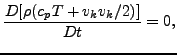(26)

along a stream line (recall that the meaning of the total derivative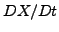is the change of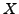following a particle), or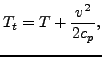(27)

where v is the magnitude of the velocity. The Mach number is defined by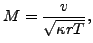(28)

where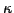is the specific heat ratio and the denominator is the speed of sound. Therefore, the total temperature satisfies: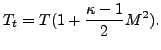(29)

The total pressure is defined as the pressure which is attained by slowing down the fluid flow in an isentropic way, i.e. a reversible adiabatic way. An ideal gas is isentropic if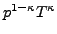is constant, which leads to the relationship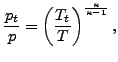(30)

and consequently to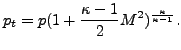(31)

Substituting the definition of mass flow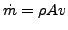, where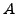is the cross section of the fluid channel, in the definition of the Mach number (and using the ideal gas equation to substitute) leads to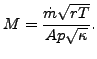(32)

Expressing the pressure and temperature as a function of the total pressure and total temperature, respectively, finally leads to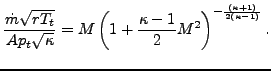(33)

This is the general gas equation, which applies to all types of flow for ideal gases. The left hand side is called the corrected flow. The right hand side exhibits a maximum for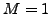, i.e. sonic conditions.

It is further possible to derive general statements for isentropic flow through network elements. Isentropic flow is reversible adiabatic by definition. Due to the adiabatic conditions the total enthalpy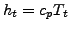is constant or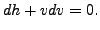(34)

The first law of thermodynamics (conservation of energy) specifies that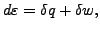(35)

or, because of the adiabatic and reversible conditions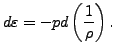(36)

Since the enthalpy, one further obtains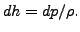(37)

Substituting this in the equation we started from leads to: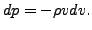(38)

The continuity equation through a network element with cross section,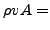constant can be written in the following differential form: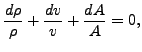(39)

or, with the equation above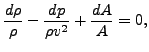(40)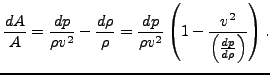(41)

Since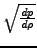is the speed of sound (use the isentropic relation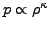and the ideal gas equationto arrive at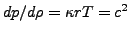), one arives at: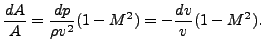(42)

Therefore, for subsonic network flow an increasing cross section leads to a decreasing velocity and an increasing pressure, whereas a decreasing cross section leads to an increasing velocity and a decreasing pressure. This is similar to what happens for incompressible flow in a tube.

For supersonic flow an increasing cross section leads to an increasing velocity and a decreasing pressure whereas a decreasing cross section leads to a decreasing velocity and an increasing pressure.

Sonic conditions can only occur if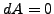, in reality this corresponds to a minimum of the cross section. Therefore, if we assume that the network elements are characterized by a uniformly increasing or decreasing cross section sonic conditions can only occur at the end nodes. This is important information for the derivation of the specific network element equations.

Using the definition of entropy per unit mass s satisfying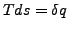and the definition of enthalpy the first law of thermodynamics for reversible processes runs like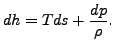(43)

Therefore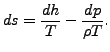(44)

.

For an ideal gas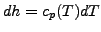andand consequently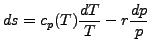(45)

or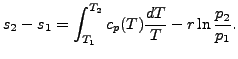(46)

Since all variables in the above equation are state variables, it also applies to irreversible processes. If the specific heat is temperature independent one obtains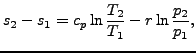(47)

linking the entropy difference between two states to the temperature and pressure difference.

Typical material properties needed for a gas network are the specific gas constant(*SPECIFIC GAS CONSTANT card), the heat capacity at constant pressureand the dynamic viscosity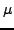(both temperature dependent and to be specified with the FLUID CONSTANTS card).

A special case is the purely thermal gas network. This applies if:

• no TYPE is specified on any *FLUID SECTION card or
• the parameter THERMAL NETWORK is used on the *STEP card or
• all mass flow is given and either all pressures or given or none.
In that case onlyis needed.

A network element is characterized by a type of fluid section. It has to be specified on the *FLUID SECTION card unless the analysis is a pure thermomechanical calculation. For gases, several types are available. At the start of the description of each type the main properties are summarized: adiabatic'' means that no heat is exchanged within the element; isentropic'' refers to constant entropy, i.e. flow without losses; symmetric'' means that the same relations apply for reversed flow; directional'' means that the flow is not allowed to be reversed.

SubsectionsNext: Orifice Up: Theory Previous: General   Contents
guido dhondt 2018-12-15